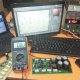Breaking News
Home / Amplifier Diagram / 1000 Watts amplifier circuit diagram pdf

# 1000 Watts amplifier circuit diagram pdf

## 1000 Watts amplifier circuit diagram pdf:

1000 Watts amplifier circuit diagram pdf, Here in this circuit is 1000 watts. in this circuit, we can use 10 transistors. TTC5200 and TTA1943. 5 are TTC5200 and another 5 are TTA1943. TTC5200 is NPN transistor and TTA1943 is PNP transistor. we know that Voltage X Amperes = Watts. So here we can use 70 voltage maximum. This transistor can take 1.3 amperes. So here in this circuit, we can use 10 transistors. then 10 X 1.3= 13 amperes. here in this circuit if we use 15 amperes and 70 voltage. then how many watts we can? as voltage X ampers = Watts then our voltage is 70 and amperes is 15. So 70 X 15= 1050 Watts. from this circuit, we can get a maximum of 1000 Watts.

The front part of this circuit,

The back part of the circuit,

This is PNG file to print out for making this circuit diagram. Please always use the original transistor. otherwise, the transistor will be no longer. there have many types of transistors quality. be sure that you are using best one.

We have to know that Voltage is important So please use a quality full transformer. and use goo diode and a minimum of 4 pieces of the capacitor. here we can use this diagram for making the strong voltage.

here in this circuit we can see that they used 8 capacitors, this capacitor values will be 100 voltage 10000 uf. and the diode will be a minimum of 20 amperes.

## 1000 Watts amplifier circuit diagram pdf:

Thanks a lot to be with us. If you want another post then please visit our website.

transistor circuit diagram of 2sa1943 and 2sc5200

Thanks a lot to be with us. If you want another post then please visit our website.

We have another post for you. like as repairing amplifier,